## ML Aggarwal Class 6 Solutions for ICSE Maths Model Question Paper 3

Section-A
Questions 1 to 8 are of 1 mark each.
Choose the correct answer from the given four options (1 to 8):
Question 1.
The difference between the place value and face value of 5 in the numeral 70542 is:
(a) 0
(b) 42
(c) 495
(d) 537
Solution:
Place value of 5
70540
500
Face value of 5
70542
5
Difference = 500 – 5 = 495 (c)

Question 2.
he sum of the successor of 99 and the predecessor of 101 is
(a) 198
(b) 200
(c) 201
(d) 199
Solution:
Successor of 99 = 100
Predecessor of 101 = 100
∴ Sum = 100 + 100 = 200 (b)

Question 3.
If x and y are two co-prime numbers, then their LCM isSolution:Question 4.
Which of the following statement is true?
(a) |15 – 6| = |15| + |—6|
(b) Additive inverse of-3 is 3
(c) -1 lies on the right of 0 on the number line
(d) -4 is greater than -3
Solution:
Additive inverse of -3 is 3 (b)

Question 5.
The fraction equivalent to $$\frac{360}{540}$$ is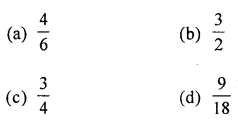Solution:
$$\frac{360}{540}$$ when divide by 90 = $$\frac{360 \div 90}{540 \div 90}=\frac{4}{6}$$ (a)

Question 6.
Which of the following numbers is divisible by 6?
(a) 5372
(b) 6495
(c) 7632
(d) 7568
Solution:
The given number = 7632
It’s unit digit = 2
So, it is divisible by 2
Sum of its digits = 7 + 6 + 3 + 2 = 18
Which is divisible by 3
∴ 7632 is divisible by both 2 and 3.
Hence it is divisible by 6 (c)

Question 7.
5 kg 5g is equal to
(a) 5.5 kg
(b) 5.05 kg
(c) 5.005 kg
(d) 5.0005 kg
Solution:
5 kg + $$\frac{5}{1000}$$ kg = 5 kg + 0.005 kg = 5.005 kg

Question 8.
The ratio of number of girls to the number of boys in a class is 7 : 5. If there are 15 boys in the class, then the number of girls in the class is
(a) 14
(b) 21
(c) 28
(d) 36
Solution:
Let number of girls = x
As per question,
7 : 5 = x : 15
$$\frac{7}{5}=\frac{x}{15}$$
x = $$\frac{7}{5}$$ × 15 = 21 girls

Section-B
Questions 9 to 14 are of 2 marks each.
Question 9.
Write the greatest and the smallest 4-digit numbers using four different digits with the condition that the digit 4 occurs at tens place.
Solution:
9847; 1042

Question 10.
Find the prime factorisation of 980.
Solution:
980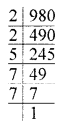∴ 980 = 2 × 2 × 5 × 7 × 7

Question 11.
Find the value of-15 – (-2) – 71 – (-8) + 6.
Solution:
-15 + 2 – 71 + 8 + 6
8 + 6 + 2 – 15 – 71 = -70

Question 12.
What fraction of the given figure is the shaded part?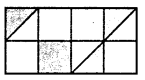Solution:
Total parts in the given figure = 16
∴ Fraction = $$\frac{5}{16}$$

Question 13.
Write the mixed fraction $$7 \frac{3}{40}$$ as a decimal number.
Solution:
Decimal number of $$7 \frac{3}{40}=\frac{283}{40}$$ = 7.075

Question 14.
The length of a pencil is 14 cm and its diameter is 7 mm. What is the ratio of the diameter of the pencil to that of its length?
Solution:
Length of a pencil = 14 cm = 140 mm
Diameter of a pencil = 7 mm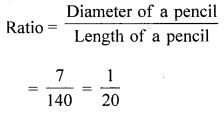Section-C
Questions 15 to 24 are of 4 marks each.
Question 15.
Estimate the product 2459 x 653 by rounding off each factor to its
(i) greatest place
(ii) nearest hundreds.
Solution:
(i) Rounding off each factor to its greatest place
∴ Product = 2400 × 600 = 1440000
(ii) nearest hundreds of 2459 is 2500 and 653 is 700
∴ Product = 2500 × 700 = 1750000

Question 16.
By using distributive laws, find 257 × 1007.
Solution:
257 × 1007
= 257 × (1000 + 7)
= 257000 + 1799
= 258799

Question 17.
Using number line, subtract (-3) from (-8).
Solution:
Start from -8 on the number line.
Move 3 units to the right. We reach at -5
∴ -8 – (-3) = -5Question 18.
Find the greatest number that will divide 76, 113 and 186 leaving remainders 4, 5 and 6 respectively.
Solution:
76 – 4 = 72
113 – 5 = 108
186 – 6 = 180
HCF of 72, 108 and 180 is 36
∴ The greatest number that will divide 72,108 and 180 is 36.

Question 19.
Arrange the following integers in descending order:
-506, 2376, 2367, -311, -509, 245.
Solution:
-506, 2376, 2367, -311, -509, 245
Descending order:
2376, 2367, 245, -311, -506, -509

Question 20.
Arrange the following fractions in ascending order $$\frac{5}{12}, \frac{1}{4}, \frac{7}{8}, \frac{5}{6}$$.
Solution:
$$\frac{5}{12}, \frac{1}{4}, \frac{7}{8}, \frac{5}{6}$$
Taking LCM of 12, 4, 8 and 6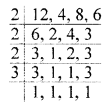= 2 × 2 × 2 × 3 = 24
Making all the fractions with denominator = 24Question 21.
Simplify: $$3 \frac{5}{6}+4 \frac{3}{4}-5-1 \frac{3}{8}$$.
Solution: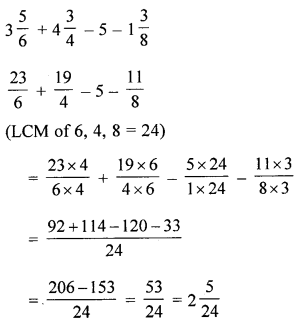Question 22.
Write all possible natural numbers using the digits 3, 0, 8. Repetition of digits is not allowed. Also find their sum.
Solution:
The given digits are 3, 0, 8 and repetition of digits is not allowed.
The one- digit numbers that can be formed are 0, 3 and 8.
We are required to write 2-digit numbers.
Out of the given digits, the possible ways of choosing the two digits are
3, 0, 3, 8; 8, 0
Using the digits 0 and 3, the numbers are 30.
Similarily, Using the digits 3 and 8, the numbers are 38 and 83.
Using the digits 8 and 0, the numbers are 80.
Hence, all possible 2-digit numbers are 30, 38, 83, 80
Now, We are required to write 3-digit numbers using the digits 0, 3, 8
and the repetition of the digits is not allowed.
Keeping 0 at unit’s place, the 3-digit number obtained are 380 and 830.
Keeping 3 at unit’s place, the 3-digit number obtained are 803.
Keeping 8 at unit’s place, the 3-digit number obtained is 308.
Hence, all possible 3-digit numbers are : 380, 830, 803, 308
All possible numbers using the digits 3, 0 and 8 are :
0, 3, 8, 30, 80, 38, 83, 380, 830, 803, 308.
∴ Sum of all possible numbers obtained using the digits 3, 0, 8
= 0 + 3 + 8 + 30 + 80 + 38 + 83 + 380 + 830 + 803 + 308 = 2563

Question 23.
Munna and Munni are aged 14 years and 10 years. Their mother wants to divide ?180 between them in the ratio of their ages. How much does each get?
Solution:
The age of Munna = 14 years
The age of Munni = 10 years
Ratio their ages = 14 : 10 = 7 : 5
Sum of their ages = 14 + 10 = 24
Share of Munna = ₹ $$\frac{14}{24}$$ × 180 = ₹175
Share of Munni = ₹$$\frac{10}{24}$$ × 180 = ₹75

Question 24.
Do the ratios 15 cm to 3 m and 15 seconds to 3 minutes form a proportion? Justify your answer.
Solution:
3 m = 3 × 100 cm = 300 cm
∴ Ratio of 15 cm to 3 m = $$\frac{15}{300}=\frac{1}{20}$$
3 minutes = 3 × 60 seconds = 180 seconds
∴ Ratio of 15 seconds to 3 minutes = $$\frac{15}{180}=\frac{1}{12}$$
As $$\frac{1}{20} \neq \frac{1}{12}$$
∴ The given ratio do not form a proportion.

Section-D
Questions 25 to 29 are of 6 marks each.
Question 25.
To stitch a shirt, 2 m 25 cm cloth is needed. Out of 30 m cloth, how many shirts can be stitched and how much cloth will remain?
Solution:
Cloth required to stitch a shirt = 2 m 25 cm
= 2 m + 25 cm
= 2 × 100 + 25 cm
= 225 cm
Total cloth available = 30 m
= 30 × 100 = 3000 cm
Shirts stitched out of 30 m = $$\frac{3000}{225}$$ cmHence 13 shirts can be stiched and 75 cm cloth will remain.

Question 26.
Four bells are ringing at intervals of 40, 30, 36 and 45 minutes respectively. At what time will they ring together again if they start ringing simultaneously at 9 A.M.?
Solution:
L.C.M. of 40, 30, 36, 45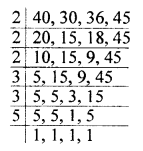∴ 2 × 2 × 2 × 3 × 3 × 5 = 360
After 360 minutes or 6 hours i.e. at 3 : 00 P.M.

Question 27.
I bought fruits worth ₹$$27 \frac{3}{4}$$ and vegetables worth ₹$$10 \frac{1}{2}$$. If I gave a fifty-rupee note to the shopkeeper, how much will I get back?
Solution: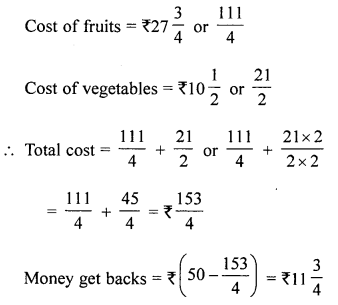Question 28.
Javed purchased vegetables weighing 10 kg. Out of this, 3 kg 450 g was potatoes, 2 kg 10 g was onions, 1 kg 750 g was tomatoes and the rest were green vegetables. What was the weight of green vegetables?
Solution:
Weight of potatoes = 3 kg 450 g = 3.450 kg
Weight of onions = 2 kg 10 g = 2.010 kg
Weight of tomatoes = 1 kg 750 g = 1.750 kg
Weight of green vegetables = x
⇒ (x + 3.450 + 2.010 + 1.750) = 10 kg
⇒ x = 2.790 kg = 2 kg 790 gm

Question 29.
To get a closer finish in a 100 m race, the runners are given different starting positions, depending upon how fast they can run. A starting position of +10 means that the runner starts 10 m in front of the starting line and -5 means that the runner starts 5 m behind the start line. The competitors starting positions are :
Abbas 0 Mohan -3 Rishi -20
Pete  + 10 Tarush + 15 Sahel + 5
(i) Who starts farthest from the finish line?
(ii) How far does Tarush have to run to reach the finish line?
(iii) How far Mohan has to run to reach the finish line?
(iv) How many metres are there between the starting positions of Rishi and Peter?
(v) What value is added if the students are asked to run 1 km daily?
Solution:
(i) Rishi starts from the farthest finish line i.e. -20 m is least.
(ii) Starting position of Tarush = +15 Total distance = 100m
Distance covered = 100 – (+15) = 100 – 15 = 85 m
(iii) Position of Mohan = -3 m by Tarush to run to reach the finish line
Total distance he has to cover is = 3 m + 100 m = 103 m
(iv) Starting position of Mike = -20 m
Starting position of Peter = +10 m
Their difference = +10 – (-20) = 30 m
(v) Good for health.